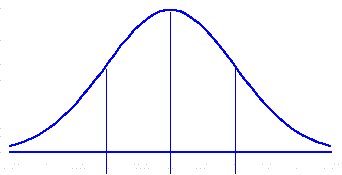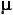Standard Error of the Difference Between the Means of Two Samples
 The logic and computational details of this procedure are described in Chapter 9 of Concepts and Applications.

The difference between the means of two samples, A and B, both randomly drawn from the same normally distributed source population, belongs to a normally distributed sampling distribution whose overall mean is equal to zero and whose standard deviation ("standard error") is equal to

square.root[(sd2/na) + (sd2/nb)]

where

sd2 = the variance of the source population (i.e., the square of the standard deviation);
na = the size of sample A;and
nb = the size of sample B.

To calculate the standard error of any particular sampling distribution of sample-mean differences, enter the mean and standard deviation (sd) of the source population, along with the values of na and nb, and then click the "Calculate" button.-1sd mean +1sd<== sourcepopulation <== samplingdistribution standard error of sample-mean differences = ±
 sd of source population sd = ± size of sample A = size of sample B =

 Home Click this link only if you did not arrive here via the VassarStats main page.